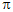# Electrical Engineering - Alternating Current and Voltage - Discussion

### Discussion :: Alternating Current and Voltage - General Questions (Q.No.3)

3.

How many degrees are there in/3 rad?

 [A]. 6° [B]. 60° [C]. 180° [D]. 27°

Explanation:

No answer description available for this question.

 Sankari said: (Jan 20, 2011) 180deg/3 = 60 deg

 Ganeshbabu said: (Jul 26, 2011) I need a formula for rad to deg.

 Ananth said: (Sep 21, 2011) I need formula for the converting to radians into degrees.

 Selva said: (Oct 13, 2011) I told you ananth. 1 deg=pi/180 rad, 1 rad=180/pi deg here given as pi/3 radians. So 1 rad=180/pi deg,then pi/3 rad=(180/pi)*(pi/3) which results in 180/3=60degree. Now its clear ma anand..

 Kumar said: (Nov 12, 2011) Degree=radians*180/pi.

 Vaishak said: (Jun 6, 2012) For converting degree to rad multiply by pi/180 eg 60 deg=60*pi/180. And for rad to degree multiply by 180/pi.

 Rkm said: (Sep 5, 2013) pi radians= 180 degree. So pi/3 = 180/3 = 60 degree.

 Nikhilesh said: (Apr 28, 2014) pi = 180 degree. pi/3 rad = 180/3 = 60 degree.

 Shan said: (Nov 22, 2015) 1 degree = 180/pi. pi/3 = pi/3*180/pi. So pi/3 rad = 60 degree.

 Naeem Rind said: (Dec 26, 2015) First of all we have to keep in mind that: 180 degrees = Pi Radians. So, Pi/3 radians X (180 degrees/Pi radians). It simplifies to: 180/3 radians. = 60 degrees.

 Raisa Paul said: (Apr 10, 2018) 180°/3=60°.

 Syed Sajid Hussain said: (Jul 1, 2019) 1 rad = 57.3°. π/3 rad = (π/3) x 57.3°. = (3.142/3) x 57.3°. = 60°.